# Selina Solutions Concise Maths Class 10 Chapter 4 Linear Inequations (In one variable)

A linear inequation in one variable will not have an equal to sign but instead any one of the four relations (<, >, ≥ or ≤). Students having any doubts on the concepts of class 10 Selina textbooks can quickly refer the Selina Solutions anytime. This is the best resource for any reference and helps students prepare confidently for their examinations.

This chapter contains two exercises. Algebraically solving linear inequations with the help of some rules are understood in these exercises. Students can also use the Concise Selina Solutions for Class 10 Maths Chapter 4 Linear Inequations (In one variable) PDF from the links given below for solving the exercise problems.

## Selina Solutions Concise Maths Class 10 Chapter 4 Linear Inequations (In one variable) Download PDF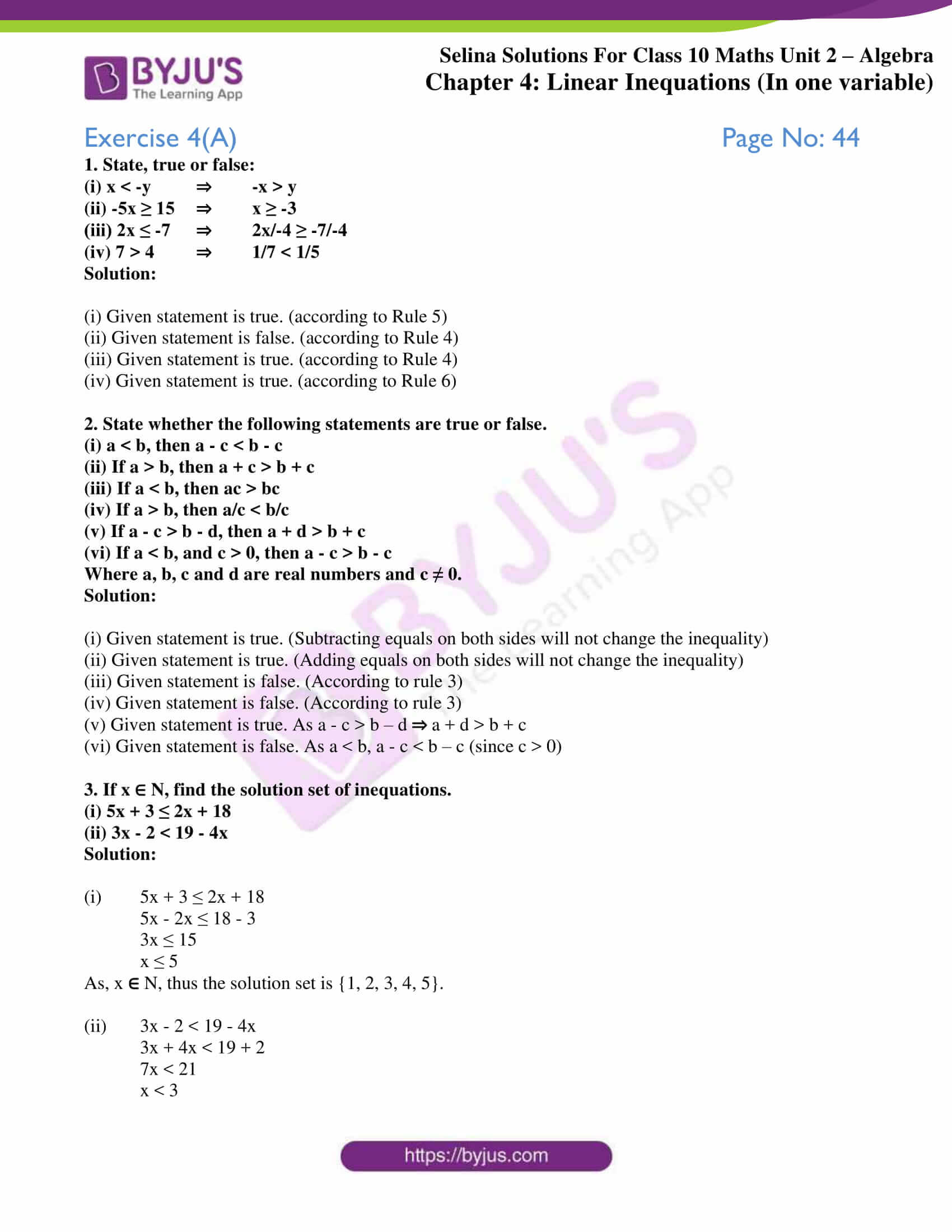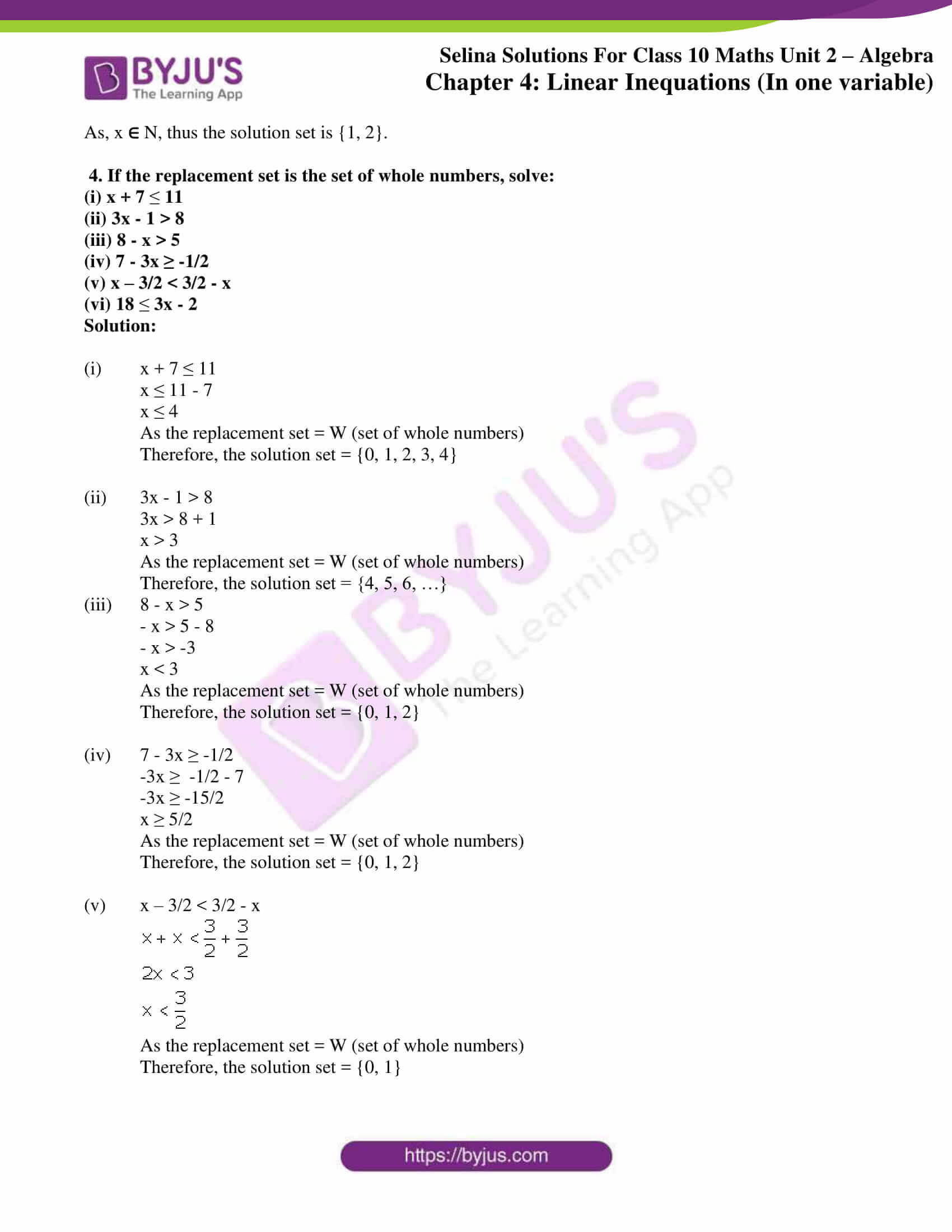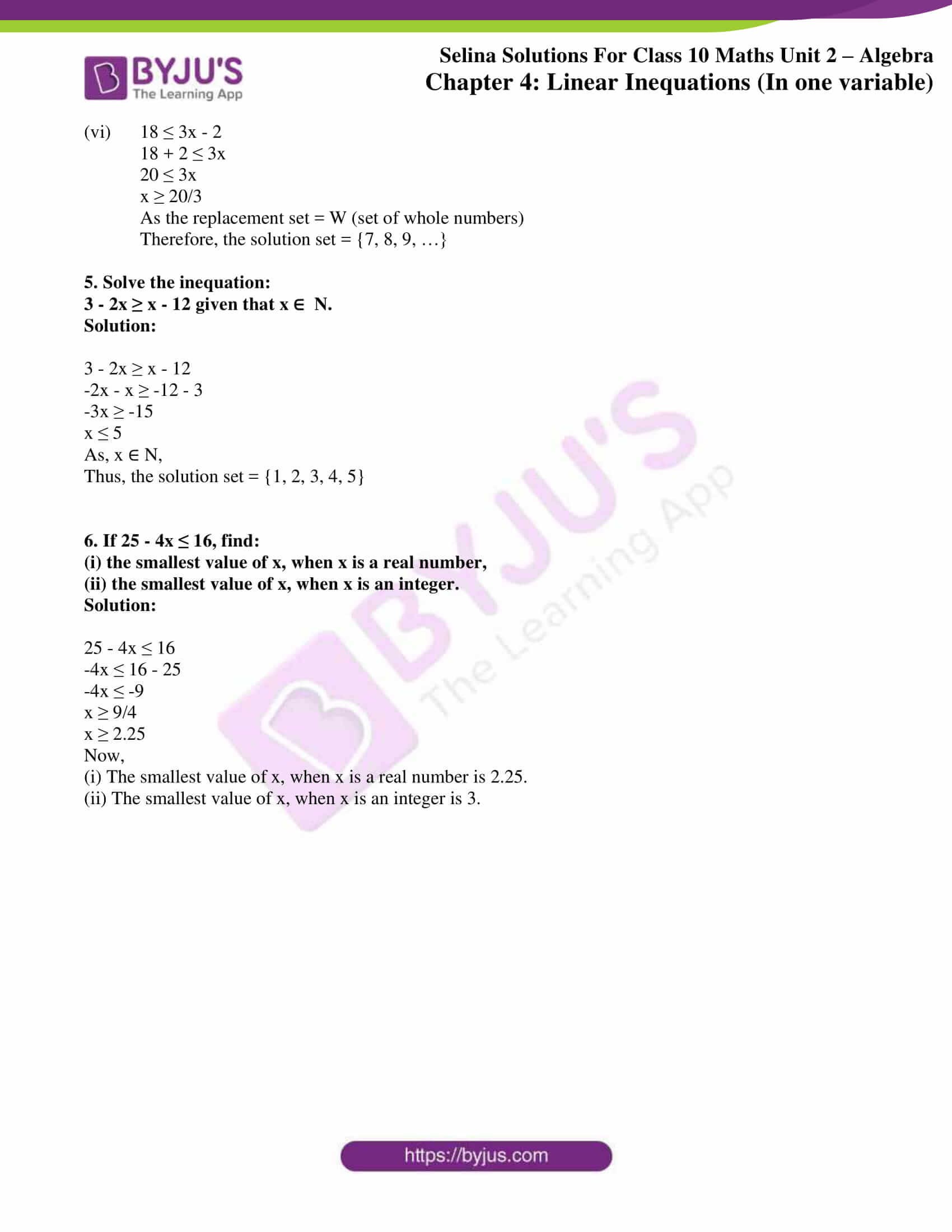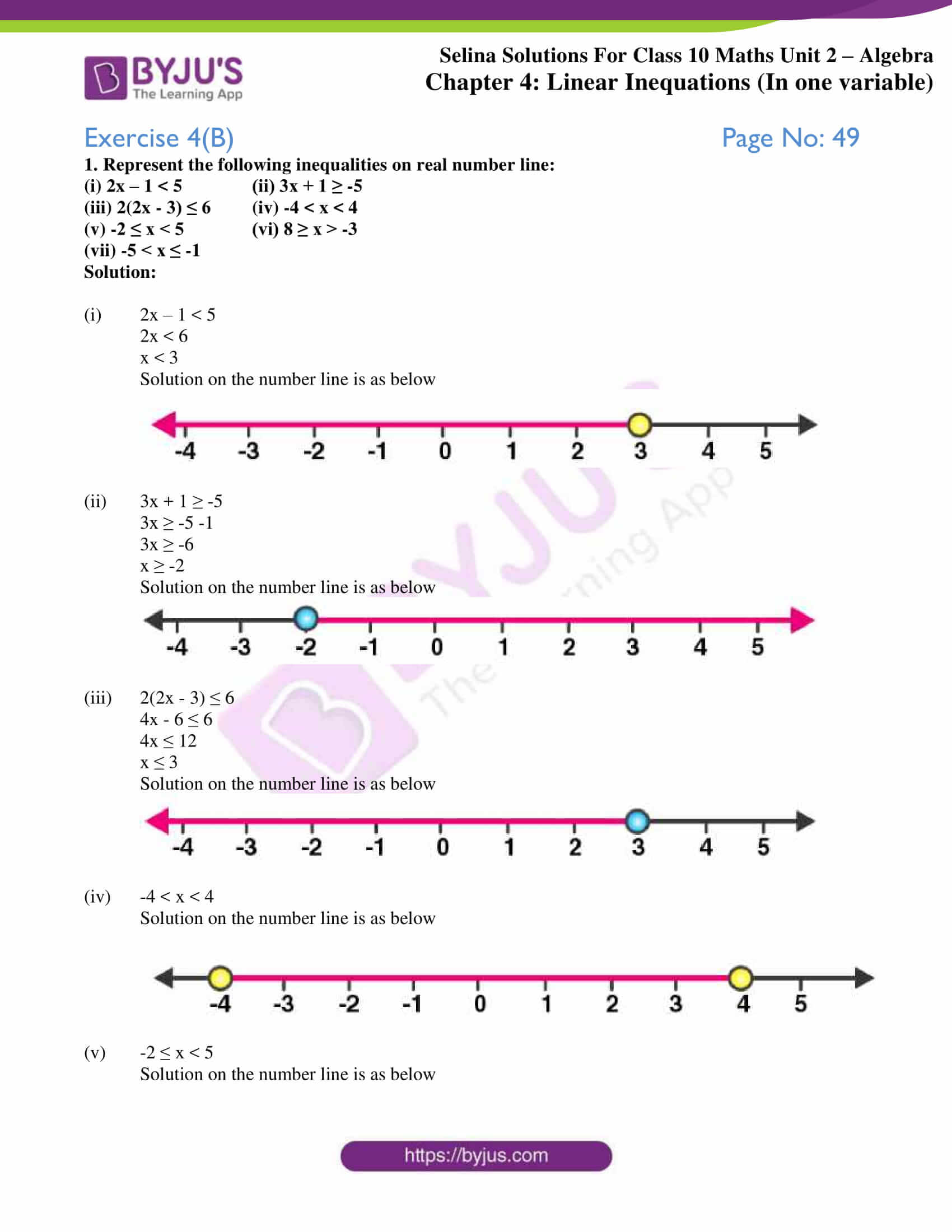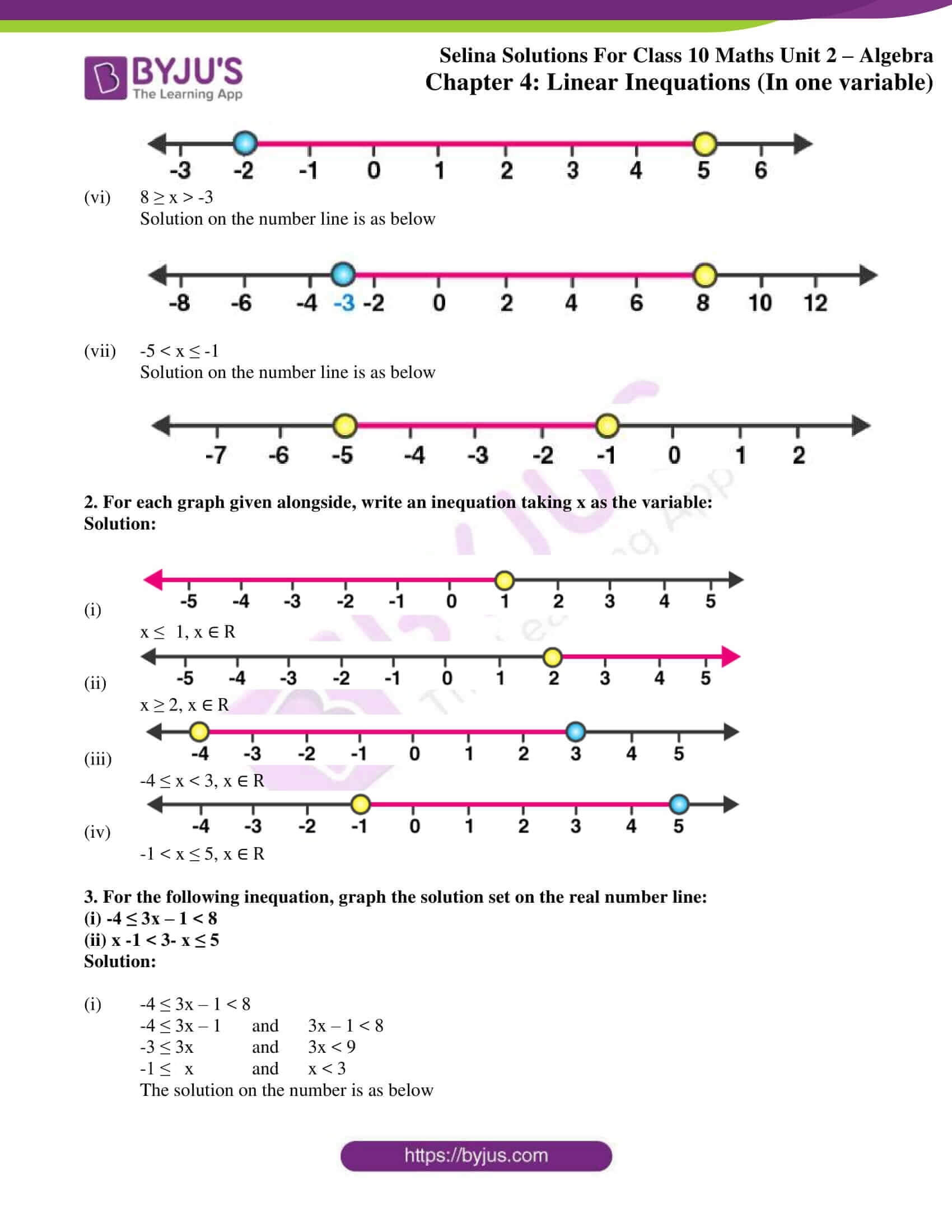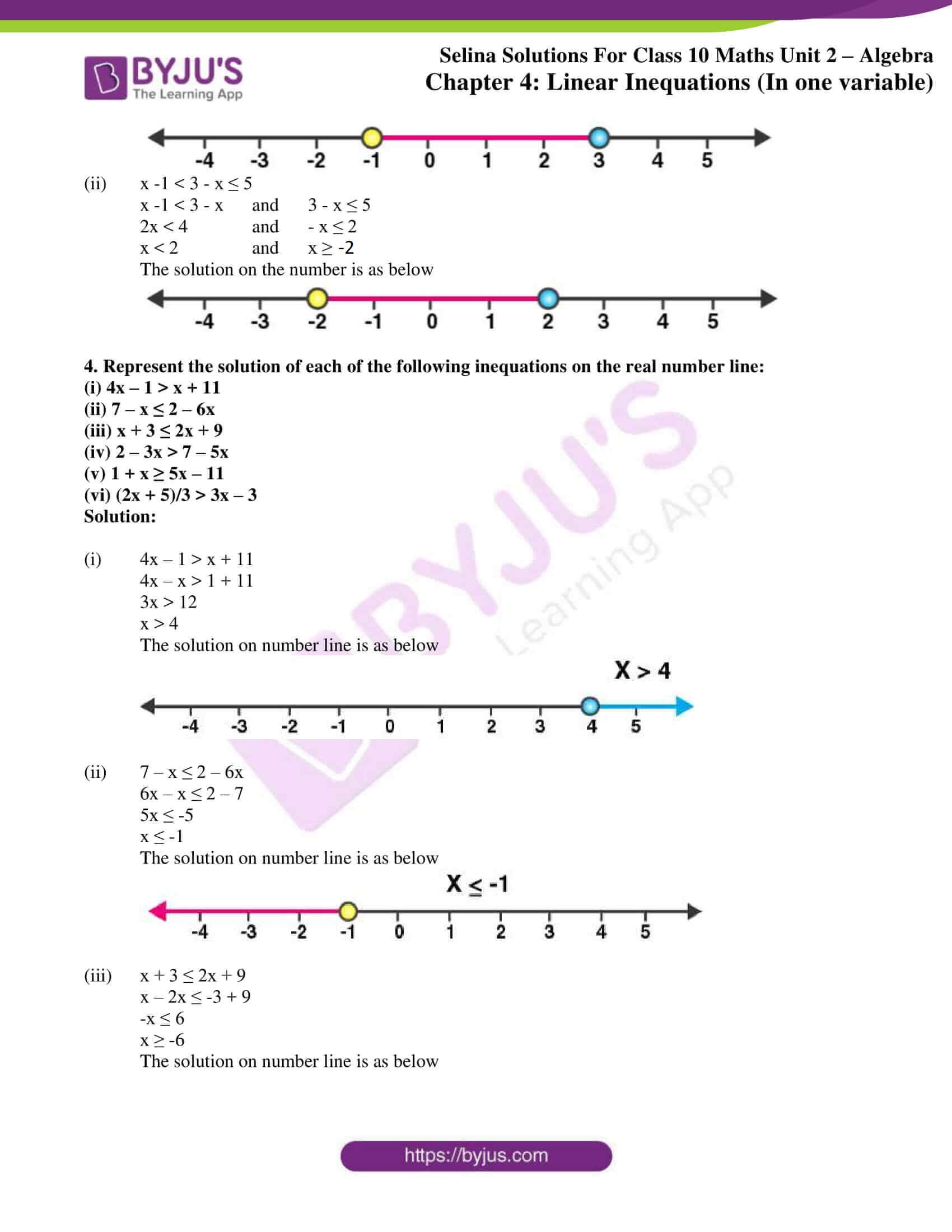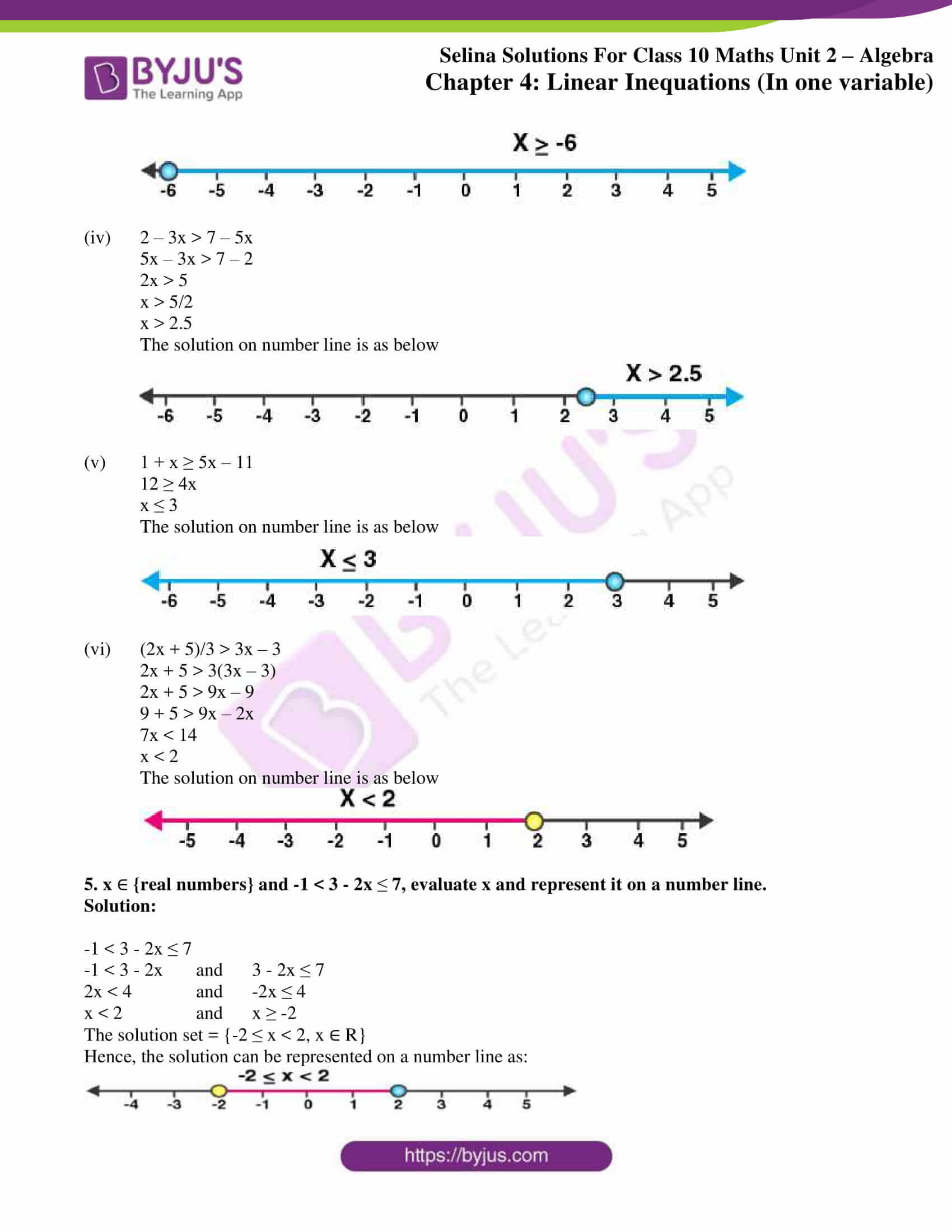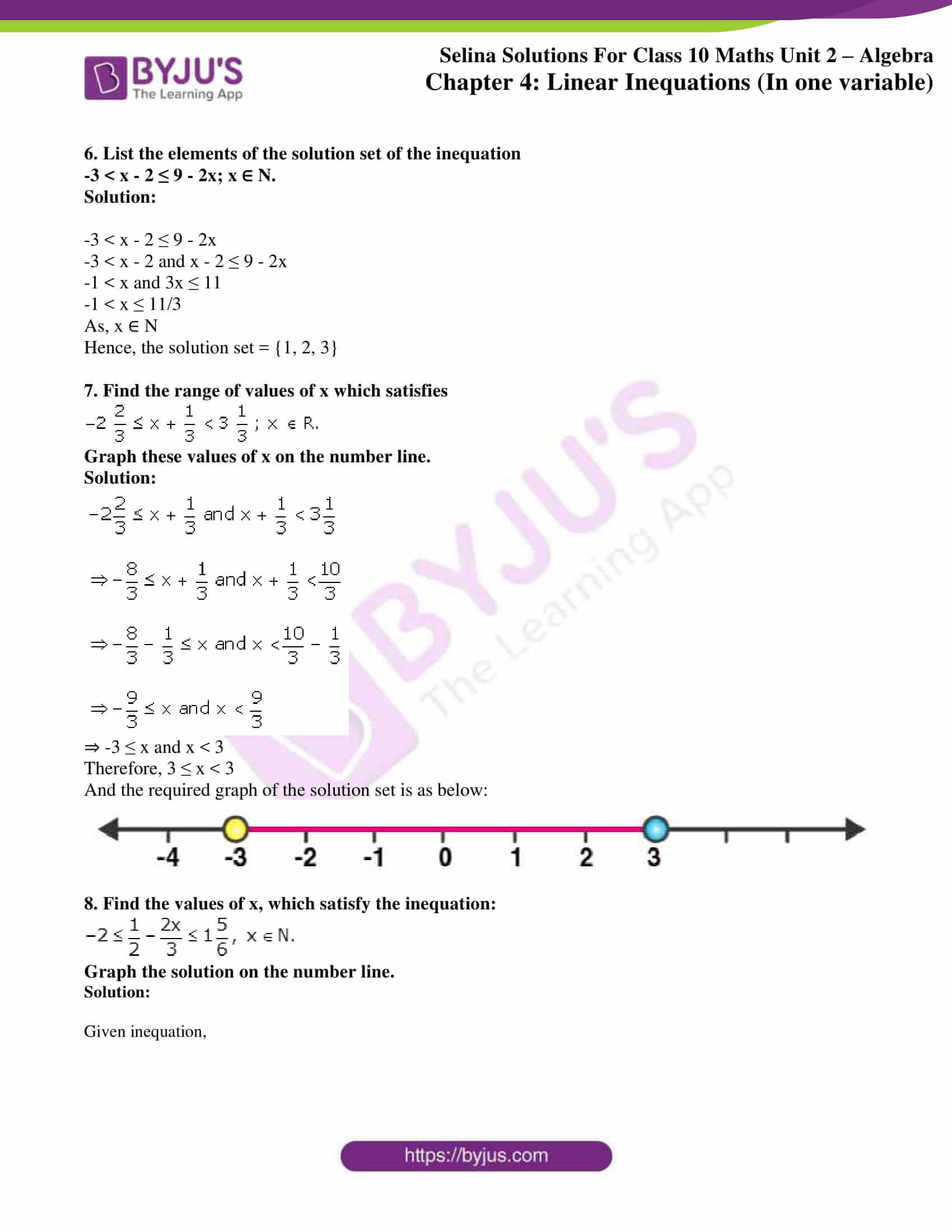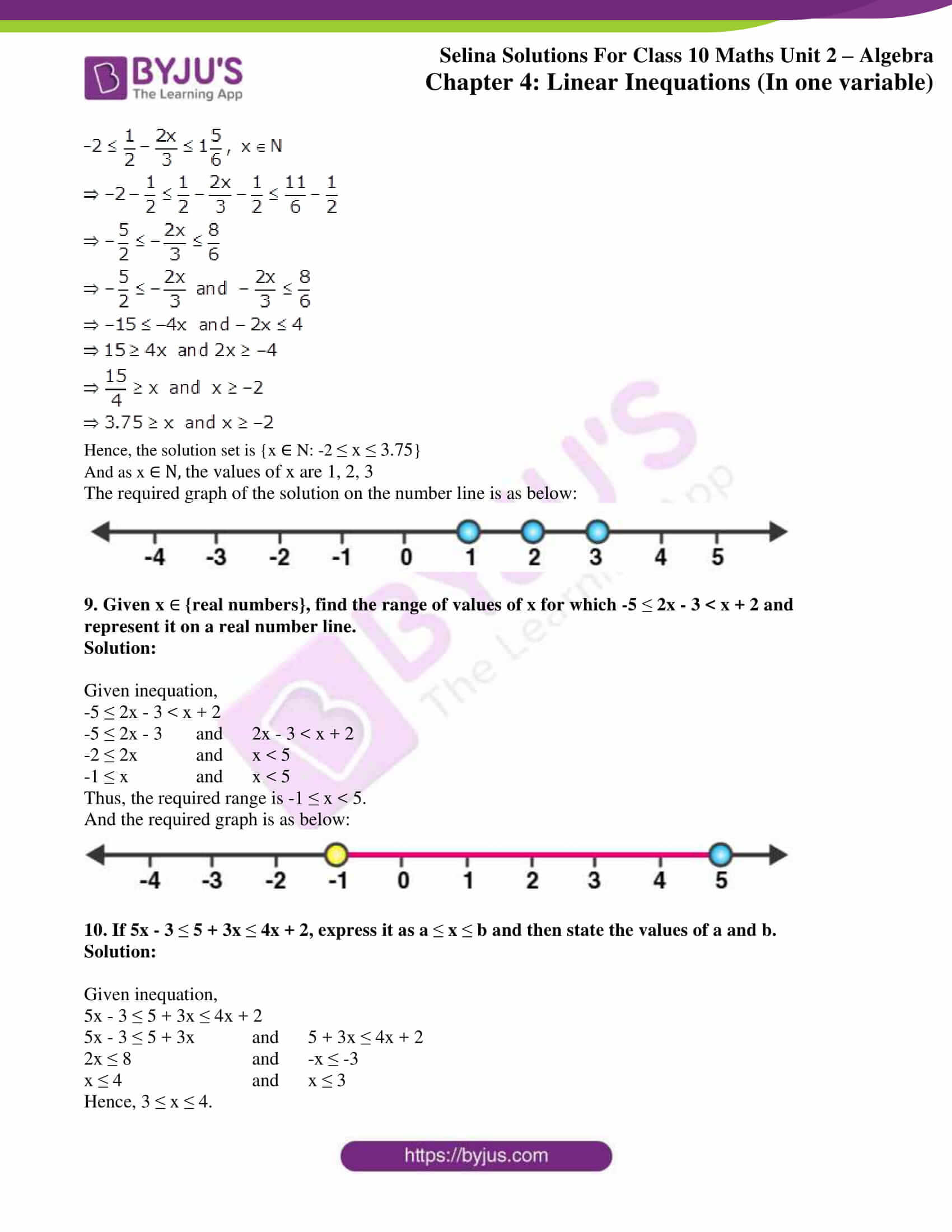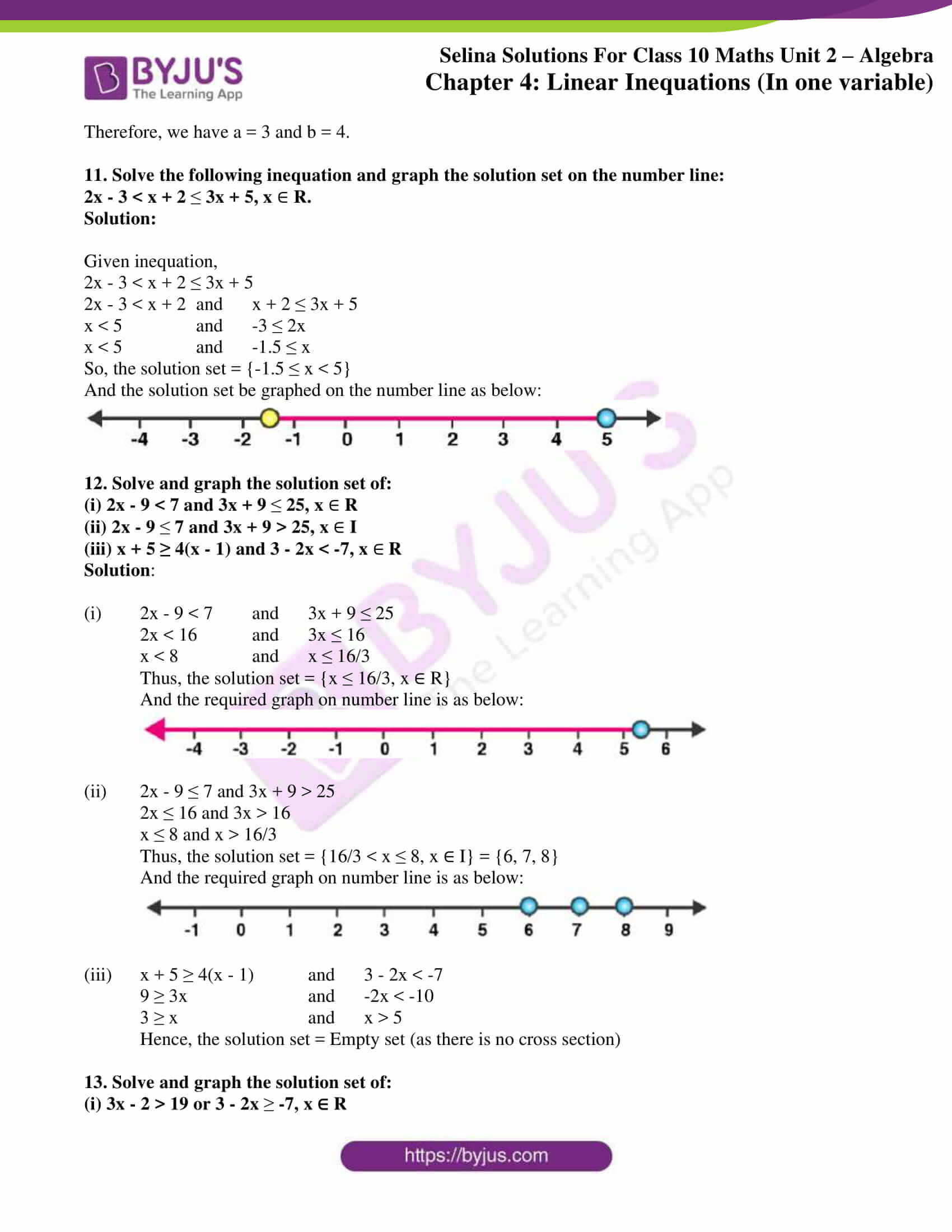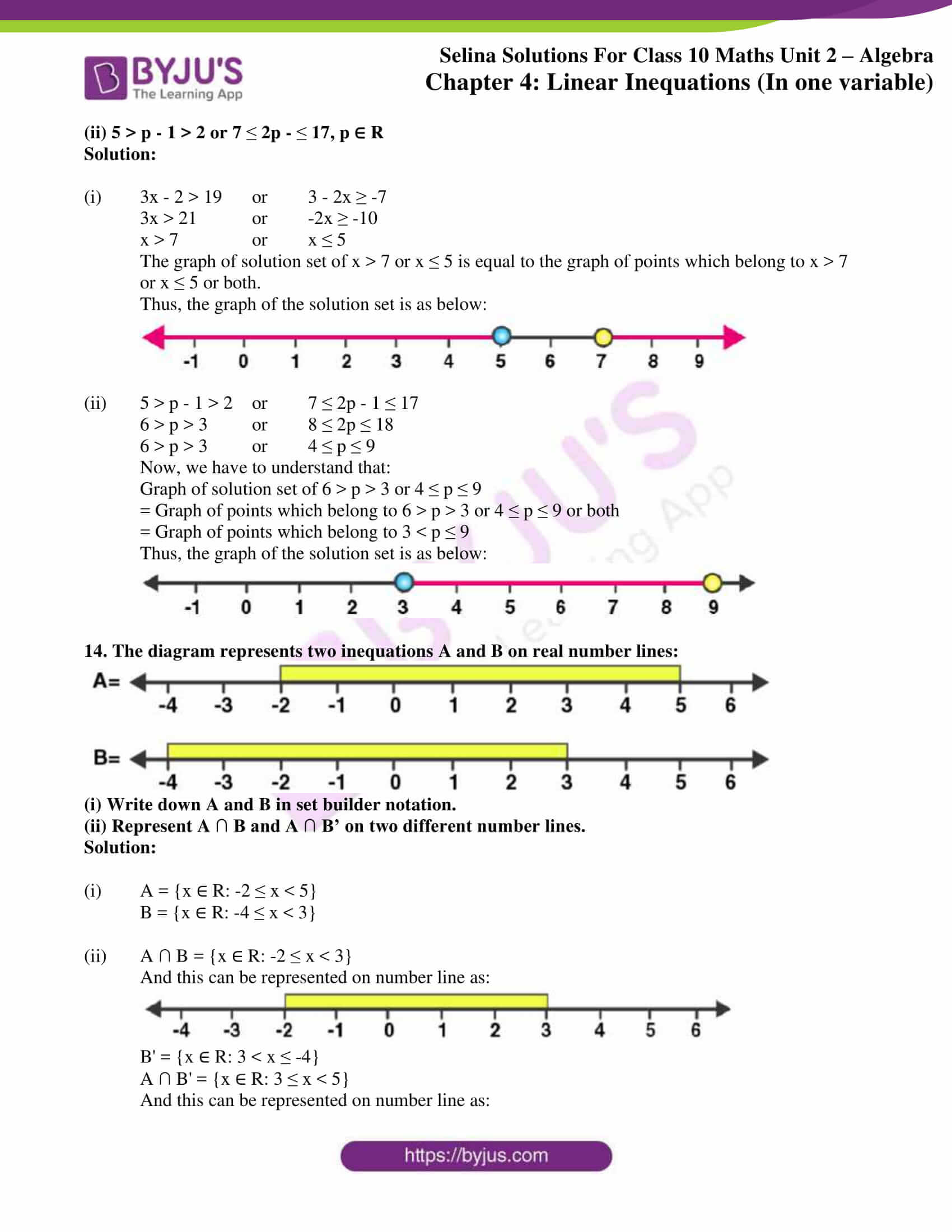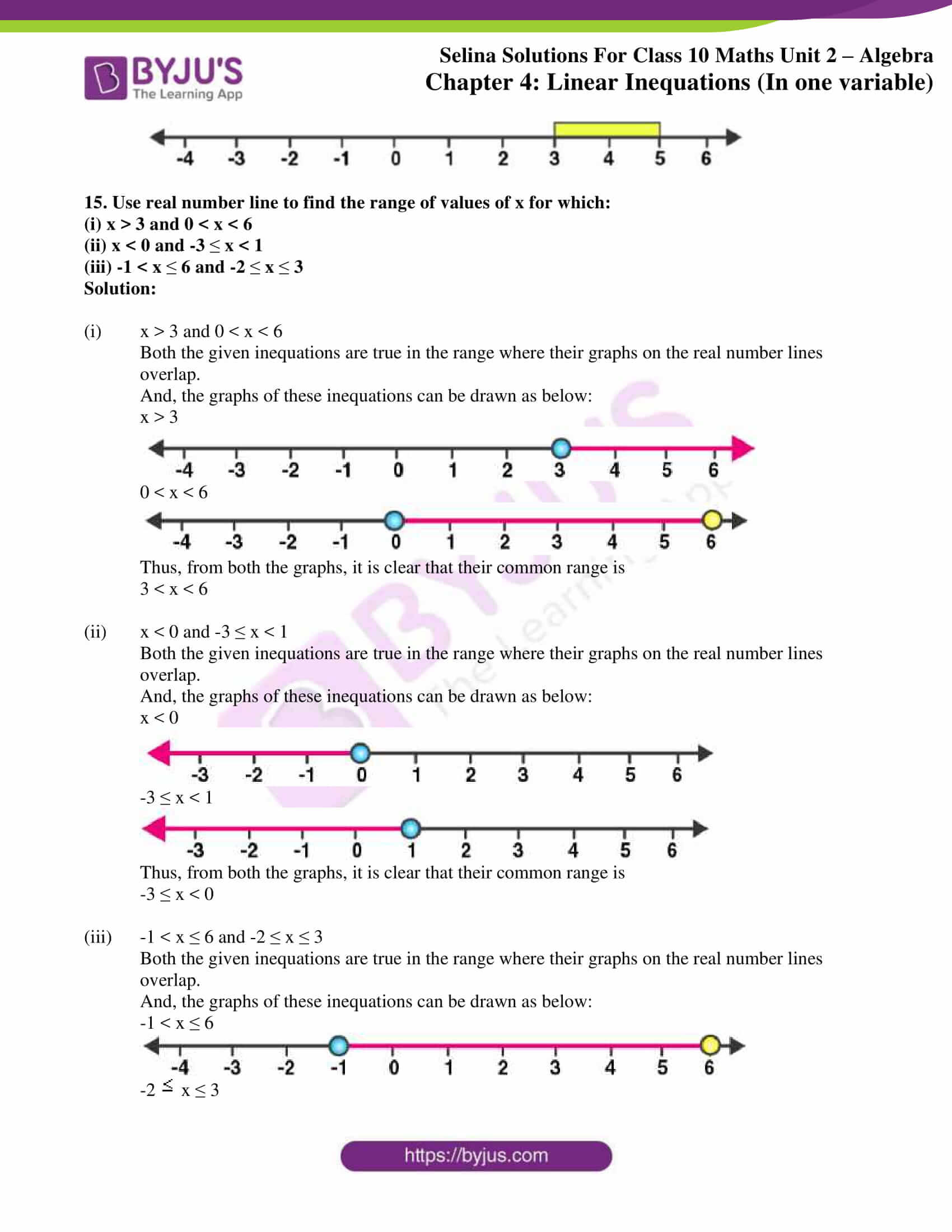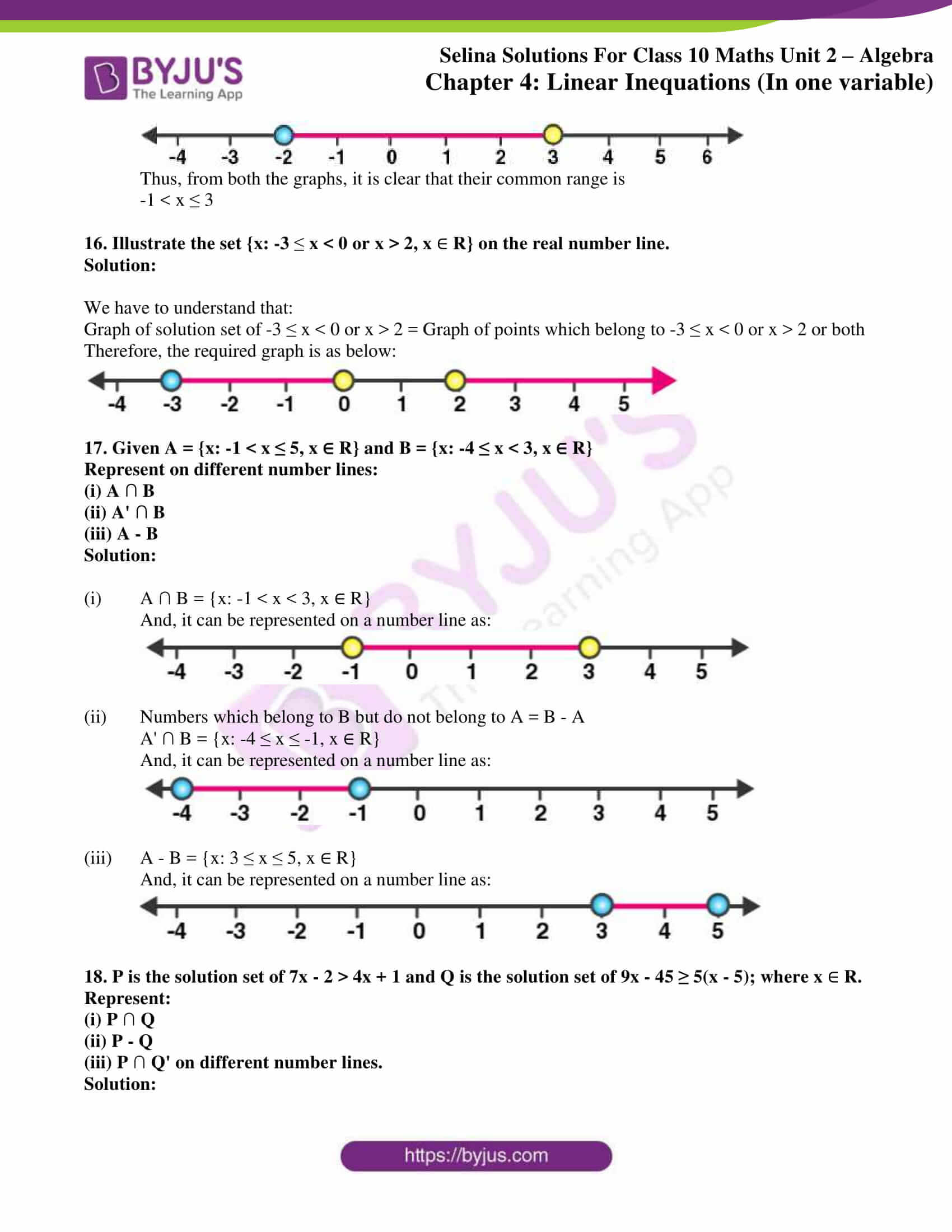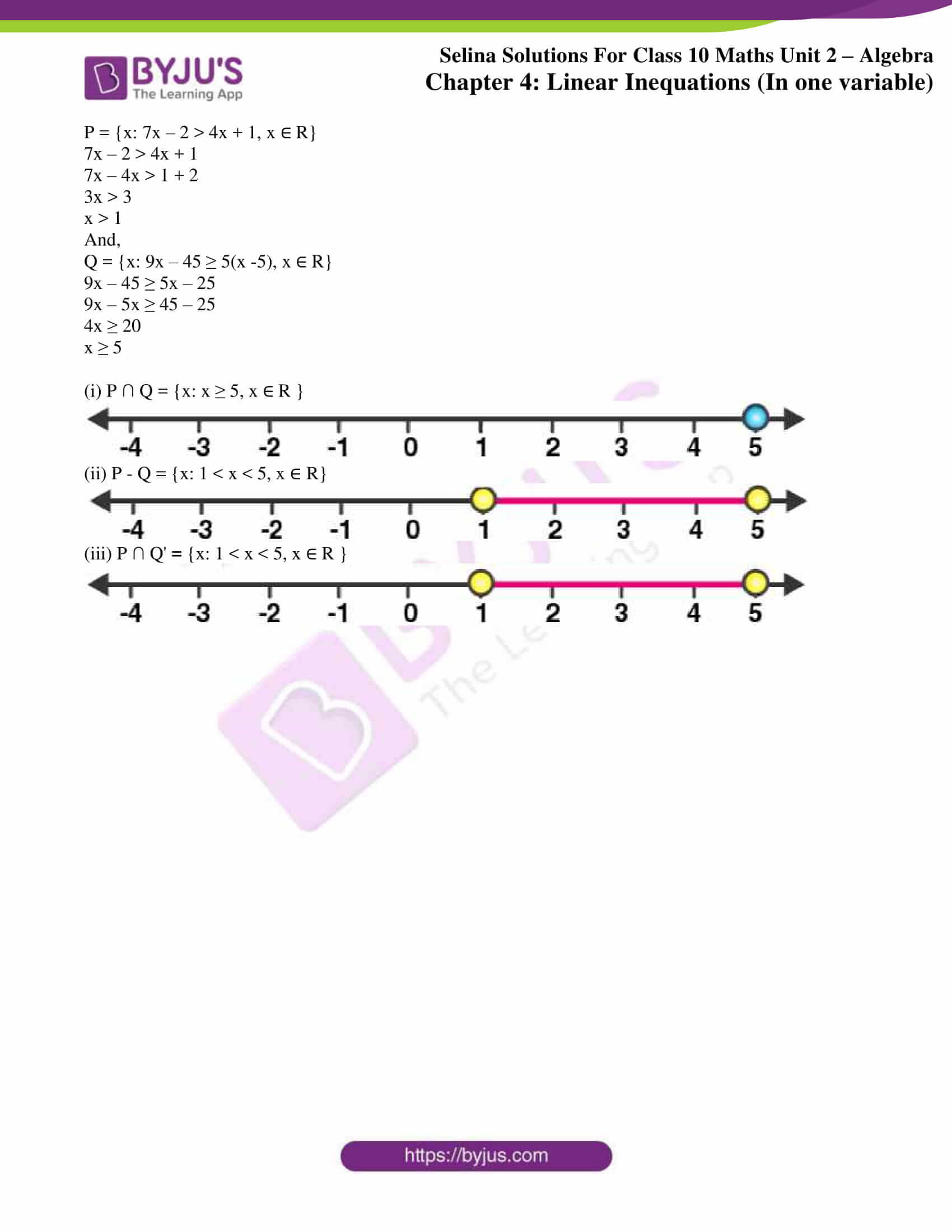### Exercises of Selina Solutions Concise Maths Class 10 Chapter 4 Linear Inequations (In one variable)

Exercise 4(A) Solutions

Exercise 4(B) Solutions

## Access Selina Solutions Concise Maths Class 10 Chapter 4 Linear Inequations (In one variable)

Exercise 4(A) Page No: 44

1. State, true or false:

(i) x < -y ⇒ -x > y

(ii) -5x ≥ 15 ⇒ x ≥ -3

(iii) 2x ≤ -7 ⇒ 2x/-4 ≥ -7/-4

(iv) 7 > 4 ⇒ 1/7 < 1/5

Solution:

(i) Given statement is true. (according to Rule 5)

(ii) Given statement is false. (according to Rule 4)

(iii) Given statement is true. (according to Rule 4)

(iv) Given statement is true. (according to Rule 6)

2. State whether the following statements are true or false.

(i) a < b, then a – c < b – c

(ii) If a > b, then a + c > b + c

(iii) If a < b, then ac > bc

(iv) If a > b, then a/c < b/c

(v) If a – c > b – d, then a + d > b + c

(vi) If a < b, and c > 0, then a – c > b – c

Where a, b, c and d are real numbers and c ≠ 0.

Solution:

(i) Given statement is true. (Subtracting equals on both sides will not change the inequality)

(ii) Given statement is true. (Adding equals on both sides will not change the inequality)

(iii) Given statement is false. (According to rule 3)

(iv) Given statement is false. (According to rule 3)

(v) Given statement is true. As a – c > b – d a + d > b + c

(vi) Given statement is false. As a < b, a – c < b – c (since c > 0)

3. If x ∈ N, find the solution set of inequations.

(i) 5x + 3 ≤ 2x + 18

(ii) 3x – 2 < 19 – 4x

Solution:

(i) 5x + 3 ≤ 2x + 18

5x – 2x ≤ 18 – 3

3x ≤ 15

x ≤ 5

As, x  N, thus the solution set is {1, 2, 3, 4, 5}.

(ii) 3x – 2 < 19 – 4x

3x + 4x < 19 + 2

7x < 21

x < 3

As, x  N, thus the solution set is {1, 2}.

4. If the replacement set is the set of whole numbers, solve:

(i) x + 7  11

(ii) 3x – 1 > 8

(iii) 8 – x > 5

(iv) 7 – 3x ≥ -1/2

(v) x – 3/2 < 3/2 – x

(vi) 18  3x – 2

Solution:

(i) x + 7 ≤ 11

x ≤ 11 – 7

x ≤ 4

As the replacement set = W (set of whole numbers)

Therefore, the solution set = {0, 1, 2, 3, 4}

(ii) 3x – 1 > 8

3x > 8 + 1

x > 3

As the replacement set = W (set of whole numbers)

Therefore, the solution set = {4, 5, 6, …}

(iii) 8 – x > 5

– x > 5 – 8

– x > -3

x < 3

As the replacement set = W (set of whole numbers)

Therefore, the solution set = {0, 1, 2}

(iv) 7 – 3x ≥ -1/2

-3x ≥  -1/2 – 7

-3x ≥ -15/2

x ≥ 5/2

As the replacement set = W (set of whole numbers)

Therefore, the solution set = {0, 1, 2}

(v)  x – 3/2 < 3/2 – x

x + x < 3/2 + 3/2

2x < 3

x < 3/2

As the replacement set = W (set of whole numbers)

Therefore, the solution set = {0, 1}

(vi) 18 ≤ 3x – 2

18 + 2 ≤ 3x

20 ≤ 3x

x ≥ 20/3

As the replacement set = W (set of whole numbers)

Therefore, the solution set = {7, 8, 9, …}

5. Solve the inequation:

3 – 2x ≥ x – 12 given that x ∈  N.

Solution:

3 – 2x ≥ x – 12

-2x – x ≥ -12 – 3

-3x ≥ -15

x ≤ 5

As, x ∈ N,

Thus, the solution set = {1, 2, 3, 4, 5}

6. If 25 – 4x ≤ 16, find:

(i) the smallest value of x, when x is a real number,

(ii) the smallest value of x, when x is an integer.

Solution:

25 – 4x ≤ 16

-4x ≤ 16 – 25

-4x ≤ -9

x ≥ 9/4

x ≥ 2.25

Now,

(i) The smallest value of x, when x is a real number is 2.25.

(ii) The smallest value of x, when x is an integer is 3.

Exercise 4(B) Page No: 49

1. Represent the following inequalities on real number line:

(i) 2x – 1 < 5 (ii) 3x + 1 ≥ -5

(iii) 2(2x – 3) ≤ 6 (iv) -4 < x < 4

(v) -2 ≤ x < 5 (vi) 8 ≥ x > -3

(vii) -5 < x ≤ -1

Solution:

(i) 2x – 1 < 5

2x < 6

x < 3

Solution on the number line is as below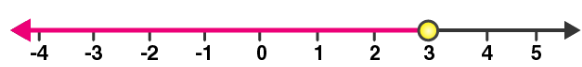(ii) 3x + 1 ≥ -5

3x ≥ -5 -1

3x ≥ -6

x ≥ -2

Solution on the number line is as below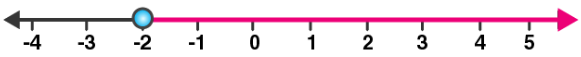(iii) 2(2x – 3) ≤ 6

4x – 6 ≤ 6

4x ≤ 12

x ≤ 3

Solution on the number line is as below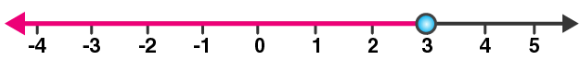(iv) -4 < x < 4

Solution on the number line is as below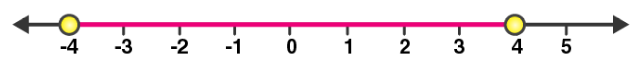(v) -2 ≤ x < 5

Solution on the number line is as below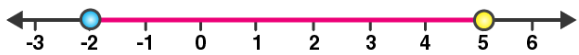(vi) 8 ≥ x > -3

Solution on the number line is as below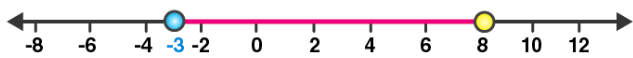(vii) -5 < x ≤ -1

Solution on the number line is as below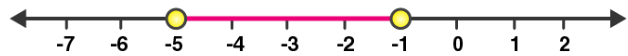2. For each graph given alongside, write an inequation taking x as the variable:

Solution:

(i)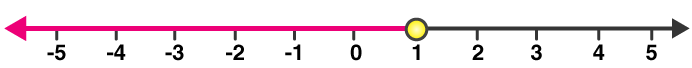x ≤ 1, x ∈ R

(ii)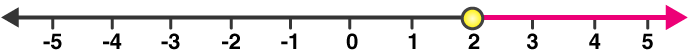x ≥ 2, x ∈ R

(iii)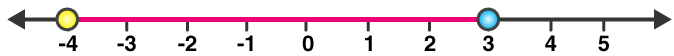-4 ≤ x < 3, x ∈ R

(iv)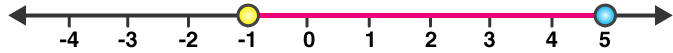-1 < x ≤ 5, x ∈ R

3. For the following inequation, graph the solution set on the real number line:

(i) -4 ≤ 3x – 1 < 8

(ii) x -1 < 3- x ≤ 5

Solution:

(i) -4 ≤ 3x – 1 < 8

-4 ≤ 3x – 1 and 3x – 1 < 8

-3 ≤ 3x and 3x < 9

-1 ≤ x and x < 3

The solution on the number is as below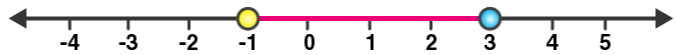(ii) x -1 < 3 – x ≤ 5

x -1 < 3 – x and 3 – x ≤ 5

2x < 4 and – x ≤ 2

x < 2 and x ≥ -2

The solution on the number is as below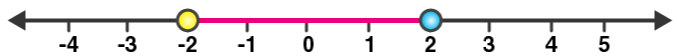4. Represent the solution of each of the following inequations on the real number line:

(i) 4x – 1 > x + 11

(ii) 7 – x ≤ 2 – 6x

(iii) x + 3 ≤ 2x + 9

(iv) 2 – 3x > 7 – 5x

(v) 1 + x ≥ 5x – 11

(vi) (2x + 5)/3 > 3x – 3

Solution:

(i) 4x – 1 > x + 11

4x – x > 1 + 11

3x > 12

x > 4

The solution on number line is as below(ii) 7 – x ≤ 2 – 6x

6x – x ≤ 2 – 7

5x ≤ -5

x ≤ -1

The solution on number line is as below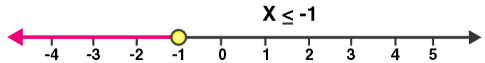(iii) x + 3 ≤ 2x + 9

x – 2x ≤ -3 + 9

-x ≤ 6

x ≥ -6

The solution on number line is as below(iv) 2 – 3x > 7 – 5x

5x – 3x > 7 – 2

2x > 5

x > 5/2

x > 2.5

The solution on number line is as below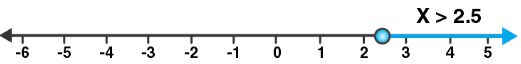(v) 1 + x ≥ 5x – 11

12 ≥ 4x

x ≤ 3

The solution on number line is as below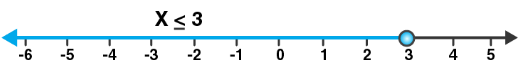(vi) (2x + 5)/3 > 3x – 3

2x + 5 > 3(3x – 3)

2x + 5 > 9x – 9

9 + 5 > 9x – 2x

7x < 14

x < 2

The solution on number line is as below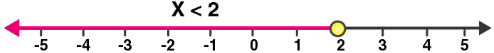5. x  {real numbers} and -1 < 3 – 2x  7, evaluate x and represent it on a number line.

Solution:

-1 < 3 – 2x ≤ 7

-1 < 3 – 2x and 3 – 2x ≤ 7

2x < 4 and -2x ≤ 4

x < 2 and x ≥ -2

The solution set = {-2 ≤ x < 2, x ∈ R}

Hence, the solution can be represented on a number line as: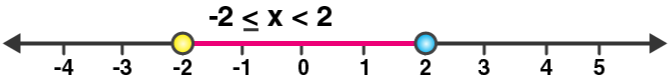6. List the elements of the solution set of the inequation

-3 < x – 2 ≤ 9 – 2x; x ∈ N.

Solution:

-3 < x – 2 ≤ 9 – 2x

-3 < x – 2 and x – 2 ≤ 9 – 2x

-1 < x and 3x ≤ 11

-1 < x ≤ 11/3

As, x ∈ N

Hence, the solution set = {1, 2, 3}

7. Find the range of values of x which satisfies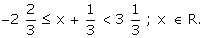Graph these values of x on the number line.

Solution: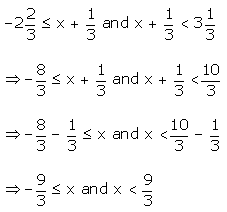⇒ -3 ≤ x and x < 3

Therefore, 3 ≤ x < 3

And the required graph of the solution set is as below: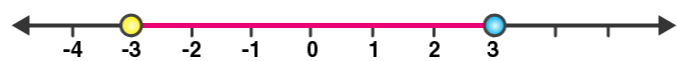8. Find the values of x, which satisfy the inequation: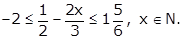Graph the solution on the number line.

Solution:

Given inequation,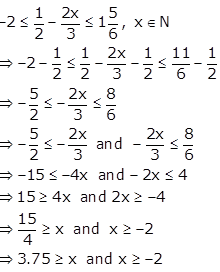Hence, the solution set is {x ∈ N: -2 ≤ x ≤ 3.75}

And as x ∈ N, the values of x are 1, 2, 3

The required graph of the solution on the number line is as below: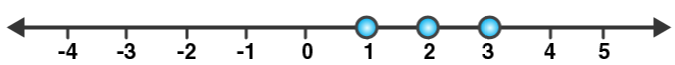9. Given x {real numbers}, find the range of values of x for which -5  2x – 3 < x + 2 and represent it on a real number line.

Solution:

Given inequation,

-5 ≤ 2x – 3 < x + 2

-5 ≤ 2x – 3 and 2x – 3 < x + 2

-2 ≤ 2x and x < 5

-1 ≤ x and x < 5

Thus, the required range is -1 ≤ x < 5.

And the required graph is as below: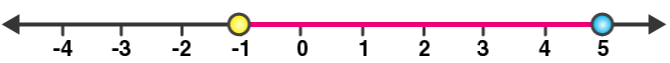10. If 5x – 3  5 + 3x  4x + 2, express it as a  x  b and then state the values of a and b.

Solution:

Given inequation,

5x – 3 ≤ 5 + 3x ≤ 4x + 2

5x – 3 ≤ 5 + 3x and 5 + 3x ≤ 4x + 2

2x ≤ 8 and -x ≤ -3

x ≤ 4 and x ≤ 3

Hence, 3 ≤ x ≤ 4.

Therefore, we have a = 3 and b = 4.

11. Solve the following inequation and graph the solution set on the number line:

2x – 3 < x + 2  3x + 5, x  R.

Solution:

Given inequation,

2x – 3 < x + 2 ≤ 3x + 5

2x – 3 < x + 2 and x + 2 ≤ 3x + 5

x < 5 and -3 ≤ 2x

x < 5 and -1.5 ≤ x

So, the solution set = {-1.5 ≤ x < 5}

And the solution set be graphed on the number line as below: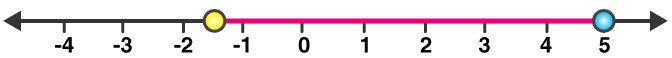12. Solve and graph the solution set of:

(i) 2x – 9 < 7 and 3x + 9  25, x  R

(ii) 2x – 9  7 and 3x + 9 > 25, x  I

(iii) x + 5 ≥ 4(x – 1) and 3 – 2x < -7, x R

Solution:

(i) 2x – 9 < 7 and 3x + 9 ≤ 25

2x < 16 and 3x ≤ 16

x < 8 and x ≤ 16/3

Thus, the solution set = {x ≤ 16/3, x ∈ R}

And the required graph on number line is as below: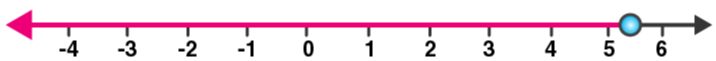(ii) 2x – 9 ≤ 7 and 3x + 9 > 25

2x ≤ 16 and 3x > 16

x ≤ 8 and x > 16/3

Thus, the solution set = {16/3 < x ≤ 8, x ∈ I} = {6, 7, 8}

And the required graph on number line is as below: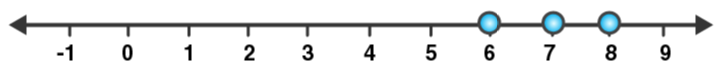(iii) x + 5 ≥ 4(x – 1) and 3 – 2x < -7

9 ≥ 3x and -2x < -10

3 ≥ x and x > 5

Hence, the solution set = Empty set (as there is no cross section)

13. Solve and graph the solution set of:

(i) 3x – 2 > 19 or 3 – 2x  -7, x ∈ R

(ii) 5 > p – 1 > 2 or 7  2p –  17, p ∈ R

Solution:

(i) 3x – 2 > 19 or 3 – 2x ≥ -7

3x > 21 or -2x ≥ -10

x > 7 or x ≤ 5

The graph of solution set of x > 7 or x ≤ 5 is equal to the graph of points which belong to x > 7 or x ≤ 5 or both.

Thus, the graph of the solution set is as below: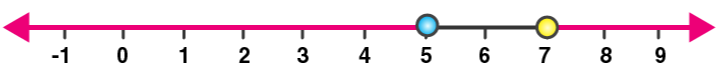(ii) 5 > p – 1 > 2 or 7 ≤ 2p – 1 ≤ 17

6 > p > 3 or 8 ≤ 2p ≤ 18

6 > p > 3 or 4 ≤ p ≤ 9

Now, we have to understand that:

Graph of solution set of 6 > p > 3 or 4 ≤ p ≤ 9

= Graph of points which belong to 6 > p > 3 or 4 ≤ p ≤ 9 or both

= Graph of points which belong to 3 < p ≤ 9

Thus, the graph of the solution set is as below:14. The diagram represents two inequations A and B on real number lines: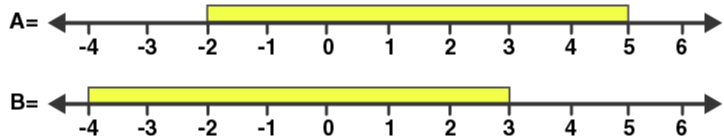(i) Write down A and B in set builder notation.

(ii) Represent A ∩ B and A ∩ B’ on two different number lines.

Solution:

(i) A = {x ∈ R: -2 ≤ x < 5}

B = {x ∈ R: -4 ≤ x < 3}

(ii) A ∩ B = {x ∈ R: -2 ≤ x < 3}

And this can be represented on number line as: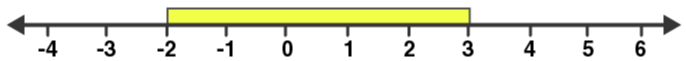B’ = {x ∈ R: 3 < x ≤ -4}

A ∩ B’ = {x ∈ R: 3 ≤ x < 5}

And this can be represented on number line as: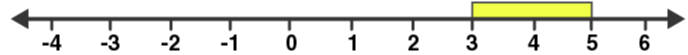15. Use real number line to find the range of values of x for which:

(i) x > 3 and 0 < x < 6

(ii) x < 0 and -3  x < 1

(iii) -1 < x  6 and -2  x  3

Solution:

(i) x > 3 and 0 < x < 6

Both the given inequations are true in the range where their graphs on the real number lines overlap.

And, the graphs of these inequations can be drawn as below:

x > 3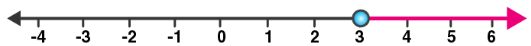0 < x < 6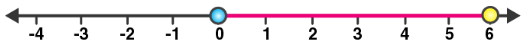Thus, from both the graphs, it is clear that their common range is

3 < x < 6

(ii) x < 0 and -3 ≤ x < 1

Both the given inequations are true in the range where their graphs on the real number lines overlap.

And, the graphs of these inequations can be drawn as below:

x < 0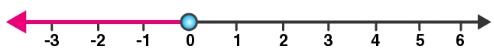-3 ≤ x < 1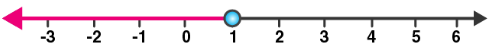Thus, from both the graphs, it is clear that their common range is

-3 ≤ x < 0

(iii) -1 < x ≤ 6 and -2 ≤ x ≤ 3

Both the given inequations are true in the range where their graphs on the real number lines overlap.

And, the graphs of these inequations can be drawn as below:

-1 < x ≤ 6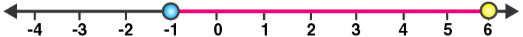-2 < x ≤ 3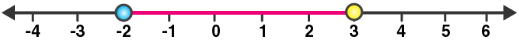Thus, from both the graphs, it is clear that their common range is

-1 < x ≤ 3

16. Illustrate the set {x: -3  x < 0 or x > 2, x  R} on the real number line.

Solution:

We have to understand that:

Graph of solution set of -3 ≤ x < 0 or x > 2 = Graph of points which belong to -3 ≤ x < 0 or x > 2 or both

Therefore, the required graph is as below: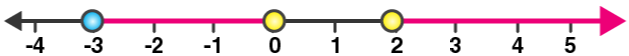17. Given A = {x: -1 < x ≤ 5, x ∈ R} and B = {x: -4 ≤ x < 3, x ∈ R}

Represent on different number lines:

(i) A ∩ B

(ii) A’ ∩ B

(iii) A – B

Solution:

(i) A ∩ B = {x: -1 < x < 3, x ∈ R}

And, it can be represented on a number line as: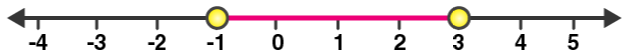(ii) Numbers which belong to B but do not belong to A = B – A

A’ ∩ B = {x: -4 ≤ x ≤ -1, x ∈ R}

And, it can be represented on a number line as: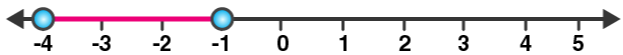(iii) A – B = {x: 3 ≤ x ≤ 5, x ∈ R}

And, it can be represented on a number line as: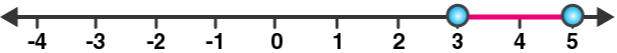18. P is the solution set of 7x – 2 > 4x + 1 and Q is the solution set of 9x – 45 ≥ 5(x – 5); where x  R. Represent:

(i) P  Q

(ii) P – Q

(iii) P  Q’ on different number lines.

Solution:

P = {x: 7x – 2 > 4x + 1, x ∈ R}

7x – 2 > 4x + 1

7x – 4x > 1 + 2

3x > 3

x > 1

And,

Q = {x: 9x – 45 ≥ 5(x -5), x ∈ R}

9x – 45 ≥ 5x – 25

9x – 5x ≥ 45 – 25

4x ≥ 20

x ≥ 5

(i) P ∩ Q = {x: x ≥ 5, x ∈ R }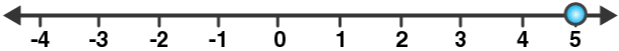(ii) P – Q = {x: 1 < x < 5, x ∈ R}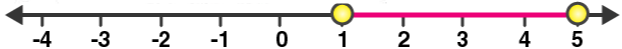(iii) P ∩ Q’ = {x: 1 < x < 5, x ∈ R }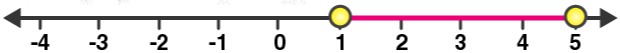The given solutions are as per the 2019-20 Concise Selina textbook. The Selina Solutions for the academic year 2020-21 will be updated soon.